Next: Problems Up: Feynman Rules for Tree Previous: Propagators

## Vertices

Spin-0: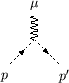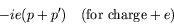(7.381)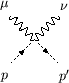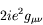(7.382)

Spin-1/2: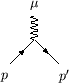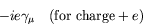(7.383)

In the last two cases, the index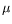has to be multiplied with that of the photon line and summed over.

A factor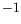for each incoming positron (outgoing electron with negative energy).

A factorin the case that two graphs differ only by the exchange of two fermions lines - this also holds for the exchange of an incoming (outgoing) particle line with an outgoing (incoming) antiparticle line, since the latter is an incoming (outgoing) particle line with negative energy.

Identical Bosons: no extra factor (according to Bose statistics).

Identical Fermions: -1 between diagram which differ only in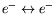or initial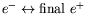.

The invariant amplitude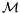is obtained by drawing all (topologically distinct and connected) Feynman diagrams for the process and assigning multiplicative factors with the various elements of each diagram.

Expressions for cross-sections are divided into two parts: first the invariant amplitude, which is a Lorentz scalar and in which physics lies, and second, the phase space and kinematical factors.

To compare with experiment one has to integrate the differential cross-section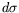over the phase-space interval which are not distinguished in the measurement. In addition one has to average over the incident polarizations and to sum over the final polarization, if these polarizations are not measured.

The invariant amplitude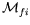can be expanded into a perturbation series in powers of the coupling constant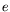using the propagator method.

The following rules allow the calculation of the expansion coefficeints. They are given below in he form which is most useful for practical calculations, namely in momentum space.Next: Problems Up: Feynman Rules for Tree Previous: Propagators
Douglas M. Gingrich (gingrich@ ualberta.ca)
2004-03-18